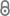# Approximate Pure Nash Equilibria in Weighted Congestion Games: Existence, Efficient Computation, and Structure

I. Caragiannis, A. Fanelli, N. Gravin, A. Skopalik, Transactions on Economics and Computation 3 (2015).320-a2-caragiannis.pdf 260.50 KB
Journal Article | English
Author
; ; ;
Abstract
We consider structural and algorithmic questions related to the Nash dynamics of weighted congestion games. In weighted congestion games with linear latency functions, the existence of pure Nash equilibria is guaranteed by a potential function argument. Unfortunately, this proof of existence is inefficient and computing pure Nash equilibria in such games is a PLS-hard problem even when all players have unit weights. The situation gets worse when superlinear (e.g., quadratic) latency functions come into play; in this case, the Nash dynamics of the game may contain cycles and pure Nash equilibria may not even exist. Given these obstacles, we consider approximate pure Nash equilibria as alternative solution concepts. A ρ--approximate pure Nash equilibrium is a state of a (weighted congestion) game from which no player has any incentive to deviate in order to improve her cost by a multiplicative factor higher than ρ. Do such equilibria exist for small values of ρ? And if so, can we compute them efficiently?We provide positive answers to both questions for weighted congestion games with polynomial latency functions by exploiting an “approximation” of such games by a new class of potential games that we call Ψ-games. This allows us to show that these games have d!-approximate pure Nash equilibria, where d is the maximum degree of the latency functions. Our main technical contribution is an efficient algorithm for computing O(1)-approximate pure Nash equilibria when d is a constant. For games with linear latency functions, the approximation guarantee is 3+√5/2 + Oγ for arbitrarily small γ > 0; for latency functions with maximum degree d≥ 2, it is d2d+o(d). The running time is polynomial in the number of bits in the representation of the game and 1/γ. As a byproduct of our techniques, we also show the following interesting structural statement for weighted congestion games with polynomial latency functions of maximum degree d ≥ 2: polynomially-long sequences of best-response moves from any initial state to a dO(d2)-approximate pure Nash equilibrium exist and can be efficiently identified in such games as long as d is a constant.To the best of our knowledge, these are the first positive algorithmic results for approximate pure Nash equilibria in weighted congestion games. Our techniques significantly extend our recent work on unweighted congestion games through the use of Ψ-games. The concept of approximating nonpotential games by potential ones is interesting in itself and might have further applications.
Publishing Year
Journal Title
Transactions on Economics and Computation
Volume
3
Issue
1
Article Number
2
LibreCat-ID

### Cite this

Caragiannis I, Fanelli A, Gravin N, Skopalik A. Approximate Pure Nash Equilibria in Weighted Congestion Games: Existence, Efficient Computation, and Structure. Transactions on Economics and Computation. 2015;3(1). doi:10.1145/2614687
Caragiannis, I., Fanelli, A., Gravin, N., & Skopalik, A. (2015). Approximate Pure Nash Equilibria in Weighted Congestion Games: Existence, Efficient Computation, and Structure. Transactions on Economics and Computation, 3(1). https://doi.org/10.1145/2614687
@article{Caragiannis_Fanelli_Gravin_Skopalik_2015, title={Approximate Pure Nash Equilibria in Weighted Congestion Games: Existence, Efficient Computation, and Structure}, volume={3}, DOI={10.1145/2614687}, number={12}, journal={Transactions on Economics and Computation}, publisher={ACM}, author={Caragiannis, Ioannis and Fanelli, Angelo and Gravin, Nick and Skopalik, Alexander}, year={2015} }
Caragiannis, Ioannis, Angelo Fanelli, Nick Gravin, and Alexander Skopalik. “Approximate Pure Nash Equilibria in Weighted Congestion Games: Existence, Efficient Computation, and Structure.” Transactions on Economics and Computation 3, no. 1 (2015). https://doi.org/10.1145/2614687.
I. Caragiannis, A. Fanelli, N. Gravin, and A. Skopalik, “Approximate Pure Nash Equilibria in Weighted Congestion Games: Existence, Efficient Computation, and Structure,” Transactions on Economics and Computation, vol. 3, no. 1, 2015.
Caragiannis, Ioannis, et al. “Approximate Pure Nash Equilibria in Weighted Congestion Games: Existence, Efficient Computation, and Structure.” Transactions on Economics and Computation, vol. 3, no. 1, 2, ACM, 2015, doi:10.1145/2614687.
Main File(s)
File Name
320-a2-caragiannis.pdf 260.50 KB
Access LevelClosed Access